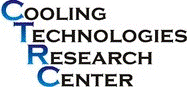## CTRC Research Publications

#### Abstract

Flows in porous media may be modeled using two major classes of approaches: (a) a macroscopic approach, where volume-averaged semiempirical equations are used to describe flow characteristics, and (b) a microscopic approach, where small-scale flow details are simulated by considering the specific geometry of the porous medium. In the first approach, small-scale details are ignored and the information so lost is represented in the governing equations using an engineering model. In the second, the intricate geometry of the porous structures is accounted for and the transport through these structures computed. The latter approach is computationally expensive if the entire physical domain were to be simulated. Computational time can be reduced by exploiting periodicity when it exists. In the present work we carry out a direct simulation of the transport in an open-cell metal foam using a periodic unit cell. The foam geometry is created by assuming the pore to be spherical. The spheres are located at the vertices and at the center of the unit cell. The periodic foam geometry is obtained by subtracting the unit cell cube from the spheres. Fluid and heat flow are computed in the periodic unit cell. Our objective in the present study is to obtain the effective thermal conductivity, pressure drop, and local heat transfer coefficient from a consistent direct simulation of the open-cell foam structure. The computed values compare well with the existing experimental measurements and semiempirical models for porosities greater than 94%. The results and the merits of the present approach are discussed.

2006

#### DOI

10.1115/1.2227038

COinS